# No Pain, High Gain: Building a Low Noise Instrumentation Amplifier with Nanovolt Sensitivity

By Moshe Gerstenhaber, Rayal Johnson, and Scott Hunt, Analog Devices

Analog Dialogue

## Introduction

Building a voltage measurement system with nanovolt sensitivity presents many design challenges. The best available op amps, such as the ultralow noise AD797, can achieve less than 1 nV/√Hz noise at 1 kHz, but the nature of low frequency noise limits the achievable noise to about 50 nV p-p over a 0.1 Hz to 10 Hz band. Oversampling and averaging can reduce the rms contribution from flat band noise at the expense of a higher data rate and additional power, but oversampling does not reduce the noise spectral density and it has no effect on noise in the 1/f region. Furthermore, large front-end gain is needed to avoid noise contribution from subsequent stages, reducing system bandwidth. Without isolation, any ground bounce or interference will also appear at the output, where it may overwhelm the low intrinsic noise of the amplifier and its input signal. A well behaved low noise instrumentation amplifier (in-amp) simplifies the design and construction of such a system, and reduces residual errors due to common-mode voltage, power supply fluctuations, and temperature drift.

The AD8428 low noise in-amp provides a precise gain of 2000 and has all of the features required to solve these problems. With 5 ppm/°C max gain drift, 0.3 μV/°C max offset voltage drift, 140 dB min CMRR to 60 Hz (120 dB min to 50 kHz), 130 dB min PSRR, and a 3.5 MHz bandwidth, the AD8428 is ideally suited to low level measurement systems. Most significantly, the 1.3 nV/√Hz voltage noise at 1 kHz and the industry-best 40 nV p-p noise from 0.1 Hz to 10 Hz enable high signal-to-noise ratio with very small signals. Two additional pins enable designers to change the gain or add a filter to reduce the noise bandwidth. These filter pins also provide a unique way to reduce the noise.

## Using Multiple AD8428 In-Amps to Lower System Noise

Figure 1 shows a circuit configuration that further reduces the system noise. The inputs and filter pins of four AD8428s are shorted together, reducing the noise by a factor of 2.
The output can be taken from any one of the in-amps to maintain low output impedance. This circuit can be extended, dividing the noise by the square root of the number of amplifiers used.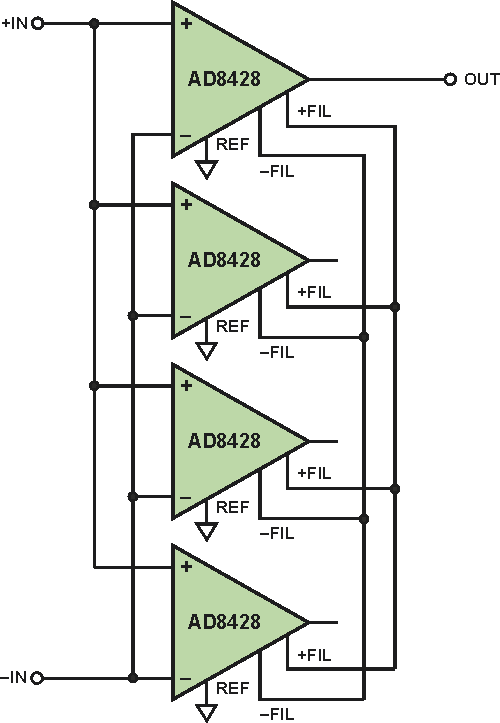Figure 1. Noise reduction circuit uses four AD8428 in-amps.

## How the Circuit Reduces Noise

The 1.3 nV/√Hz typical referred-to-input (RTI) spectral noise generated by each AD8428 is uncorrelated with the noise generated by the other amplifiers. The uncorrelated noise sources add as the root-sum-of-squares (RSS) at the filter pins. The input signal, on the other hand, is positively correlated. Each AD8428 generates the same voltage at its filter pins in response to the signal, so connecting multiple AD8428s doesn’t change the voltage, and the gain remains 2000.

## Noise Analysis

The following analysis of the simplified version of the circuit in Figure 2 shows that two AD8428s connected this way reduces the noise by a factor of √2. The noise of each AD8428 can be modeled at its +IN pin. To determine the total noise, ground the inputs and use superposition to combine the noise sources.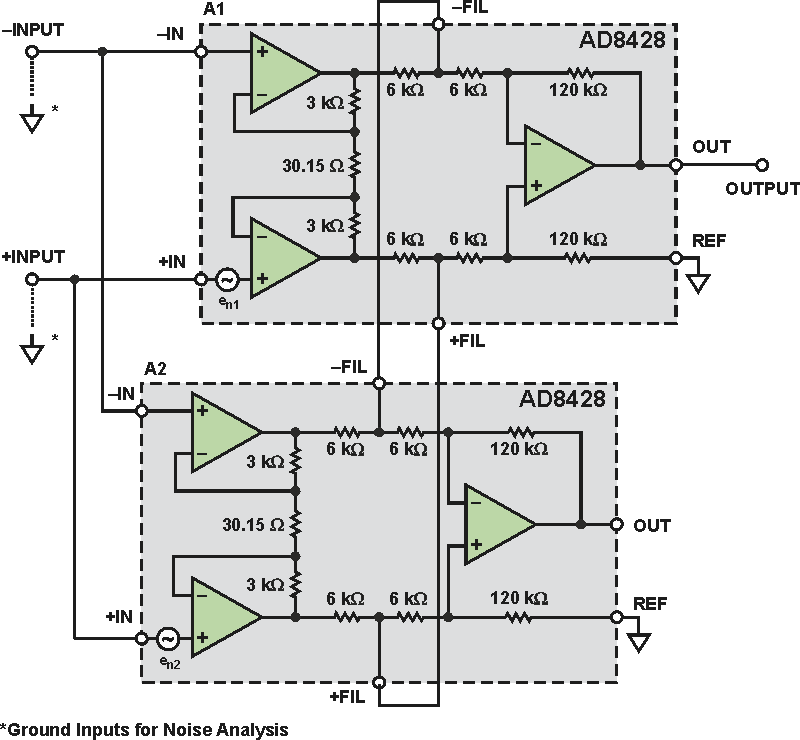Figure 2. Simplified circuit model for noise analysis.

Noise source en1 gets amplified by a differential gain of 200 to the output of preamplifier A1. For this part of the analysis, the outputs of preamplifier A2 are noiseless with grounded inputs. The 6 kΩ/6 kΩ resistor divider from each output of Preamplifier A1 to the corresponding output of Preamplifier A2 can be replaced by its Thévenin equivalent: half of the noise voltage at the output of Preamplifier A1 with a 3 kΩ series resistor. This division is the mechanism that reduces the noise. The full nodal analysis shows that the output voltage in response to en1 is 1000 × en1. By symmetry, the output voltage in response to noise voltage en2 is 1000 × en2. The magnitude of en1 and en2 are both equal to en, and will add as RSS, causing an overall output noise of 1414 × en.

In order to refer this back to the input, the gain must be verified. Assume a differential signal VIN is applied between +INPUT and –INPUT. The differential voltage at the output of the first stage of A1 will be VIN × 200. The same voltages appear at the outputs of Preamplifier A2, so there is no signal division through the 6 kΩ/6 kΩ divider, and nodal analysis shows an output of VIN × 2000. Thus, the total voltage noise RTI is en × 1414/2000, or equivalently en/√2. Using the 1.3 nV/√Hz typical noise density of the AD8428, the two amplifier configuration yields a noise density of about 0.92 nV/√Hz.

With additional amplifiers, the impedance at the filter pin changes, reducing the noise further. For example, using four AD8428s as shown in Figure 1, the 6 kΩ resistor from the preamplifier output to the filter pin is followed by three 6 kΩ resistors to each of the noiseless preamplifier outputs. This effectively creates a 6 kΩ/2 kΩ resistor divider, dividing the noise by four. The total noise of all four amplifiers then becomes en/2, as predicted.

## Making the Noise vs. Power Trade-Off

The main trade-off is power consumption vs. noise. The AD8428 has very high noise-to-power efficiency, with input noise density of 1.3 nV/√Hz for 6.8 mA maximum supply current. For comparison, consider the low noise AD797 op amp, which requires 10.5 mA maximum supply current to achieve 0.9 nV/√Hz. A discrete G = 2000, low noise in-amp built with two AD797 op amps and a low power difference amplifier could use more than 21 mA to achieve 1.45 nV/√Hz noise RTI, contributed by the two op amps and a 30.15 Ω resistor.

In addition to the power supply considerations of using many amplifiers in parallel, the designer must also consider the thermal environment. A single AD8428 with a ±5 V supply will have a temperature rise of about 8 °C due to internal power dissipation. If many devices are placed close together or in an enclosed space, they can mutually heat one another, and thermal management techniques should be considered.

## Simulation with SPICE

Although it should not be used as a substitute for prototyping, circuit simulation with SPICE can be a useful first step in validating circuit ideas such as this. To verify this circuit, the ADIsimPE simulator and the AD8428 SPICE macro model were used to simulate the circuit performance with two devices in parallel. The results shown in Figure 3 demonstrate the expected behavior: a gain of 2000 and 30% lower noise.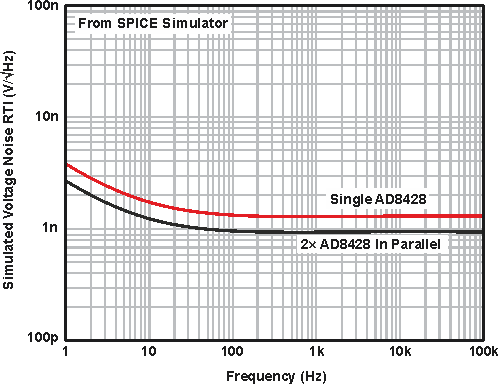Figure 3. SPICE simulation results.

## Measurement Results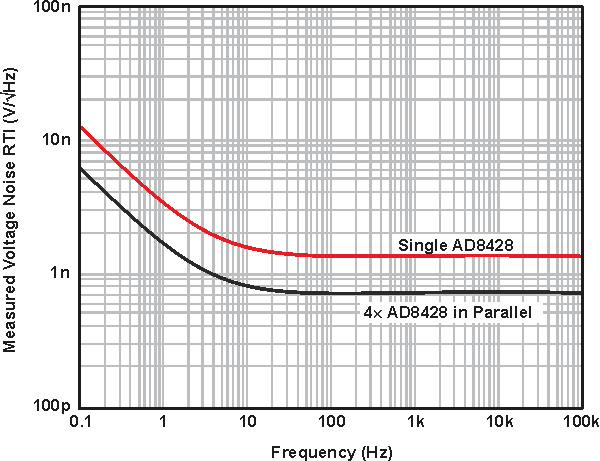Figure 4. Measured voltage noise spectrum of circuit shown in Figure 1.

The full circuit with four AD8428s was measured on the bench. The measured RTI noise has a spectral density of 0.7 nV/√Hz at 1 kHz and 25 nV p-p from 0.1 Hz to 10 Hz. This is lower than the noise of many nanovoltmeters. The measured noise spectrum and peak-to-peak noise are shown in Figure 4 and Figure 5, respectively.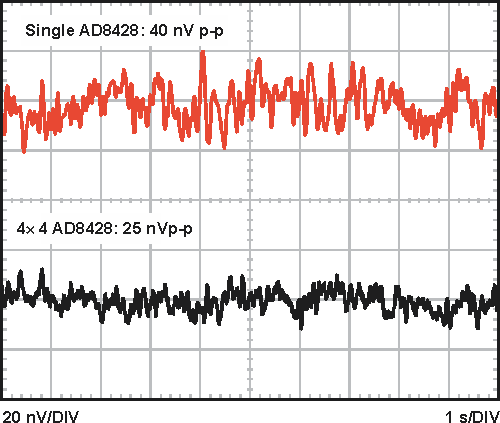Figure 5. Measured 0.1 Hz to 10 Hz RTI noise of circuit shown in Figure 1.

## Conclusion

Nanovolt sensitivity is a difficult goal that presents many design challenges. For systems that require low noise and high gain, the AD8428 in-amp has the features required to implement a high performance design. Furthermore, its unique configuration allows it to add this unusual circuit to the nanovolt toolbox.

## References

1. MT-047 Tutorial. Op Amp Noise.
2. MT-048 Tutorial. Op Amp Noise Relationships: 1/f Noise, RMS Noise, and Equivalent Noise Bandwidth.
3. MT-049 Tutorial. Op Amp Total Output Noise Calculations for Single-Pole System.
4. MT-050 Tutorial. Op Amp Total Output Noise Calculations for Second-Order System.
5. MT-065 Tutorial. In-Amp Noise.

analog.com